# 7.3 Polar coordinates  (Page 6/16)

 Page 6 / 16

Determine the symmetry of the graph determined by the equation $r=2\phantom{\rule{0.2em}{0ex}}\text{cos}\left(3\theta \right)$ and create a graph.

Symmetric with respect to the polar axis.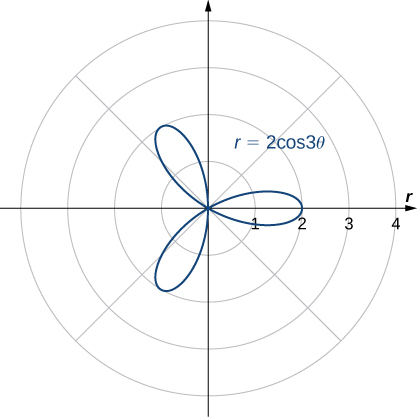## Key concepts

• The polar coordinate system provides an alternative way to locate points in the plane.
• Convert points between rectangular and polar coordinates using the formulas
$x=r\phantom{\rule{0.2em}{0ex}}\text{cos}\phantom{\rule{0.2em}{0ex}}\theta \phantom{\rule{0.2em}{0ex}}\text{and}\phantom{\rule{0.2em}{0ex}}y=r\phantom{\rule{0.2em}{0ex}}\text{sin}\phantom{\rule{0.2em}{0ex}}\theta$

and
$r=\sqrt{{x}^{2}+{y}^{2}}\phantom{\rule{0.2em}{0ex}}\text{and}\phantom{\rule{0.2em}{0ex}}\text{tan}\phantom{\rule{0.2em}{0ex}}\theta =\frac{y}{x}.$
• To sketch a polar curve from a given polar function, make a table of values and take advantage of periodic properties.
• Use the conversion formulas to convert equations between rectangular and polar coordinates.
• Identify symmetry in polar curves, which can occur through the pole, the horizontal axis, or the vertical axis.

In the following exercises, plot the point whose polar coordinates are given by first constructing the angle $\theta$ and then marking off the distance r along the ray.

$\left(3,\frac{\pi }{6}\right)$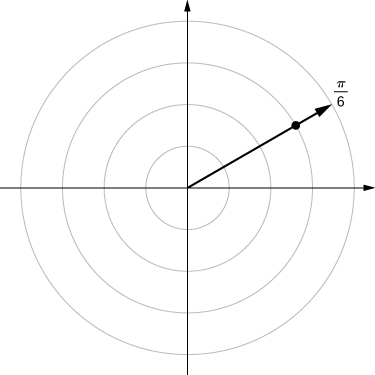$\left(-2,\frac{5\pi }{3}\right)$

$\left(0,\frac{7\pi }{6}\right)$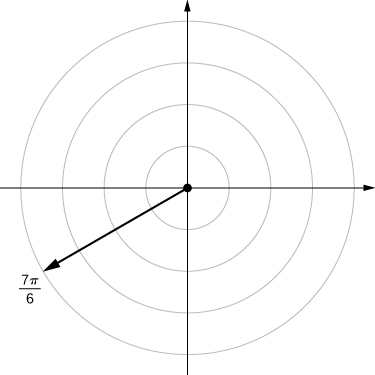$\left(-4,\frac{3\pi }{4}\right)$

$\left(1,\frac{\pi }{4}\right)$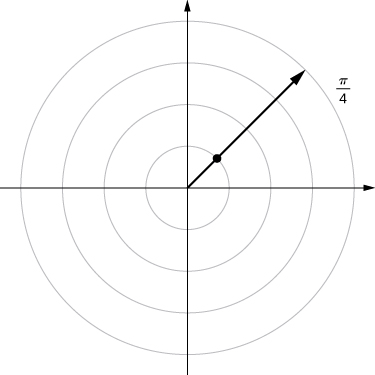$\left(2,\frac{5\pi }{6}\right)$

$\left(1,\frac{\pi }{2}\right)$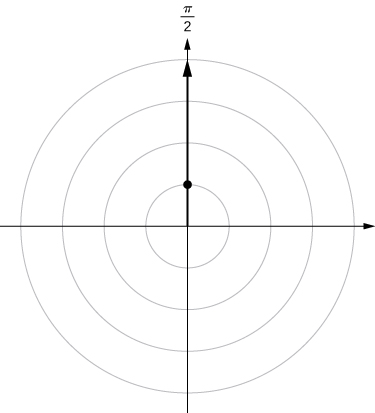For the following exercises, consider the polar graph below. Give two sets of polar coordinates for each point.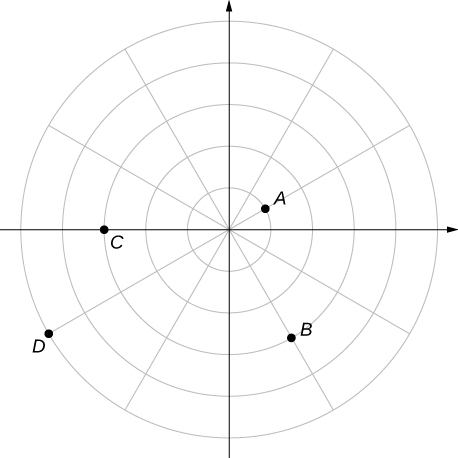Coordinates of point A .

Coordinates of point B .

$B\begin{array}{cc}\left(3,\frac{\text{−}\pi }{3}\right)\hfill & B\left(-3,\frac{2\pi }{3}\right)\hfill \end{array}$

Coordinates of point C .

Coordinates of point D .

$D\left(5,\frac{7\pi }{6}\right)D\left(-5,\frac{\pi }{6}\right)$

For the following exercises, the rectangular coordinates of a point are given. Find two sets of polar coordinates for the point in $\left(0,2\pi \right].$ Round to three decimal places.

$\left(2,\phantom{\rule{0.2em}{0ex}}2\right)$

$\left(3,-4\right)$ (3, −4)

$\begin{array}{cc}\left(5,-0.927\right)\hfill & \left(-5,-0.927+\pi \right)\hfill \end{array}$

$\left(8,\phantom{\rule{0.2em}{0ex}}15\right)$

$\left(-6,\phantom{\rule{0.2em}{0ex}}8\right)$

$\left(10,-0.927\right)\left(-10,-0.927+\pi \right)$

$\left(4,\phantom{\rule{0.2em}{0ex}}3\right)$

$\left(3,\text{−}\sqrt{3}\right)$

$\left(2\sqrt{3},-0.524\right)\left(-2\sqrt{3},-0.524+\pi \right)$

For the following exercises, find rectangular coordinates for the given point in polar coordinates.

$\left(2,\frac{5\pi }{4}\right)$

$\left(-2,\frac{\pi }{6}\right)$

$\left(\begin{array}{cc}\text{−}\sqrt{3},\hfill & -1\hfill \end{array}\right)$

$\left(5,\frac{\pi }{3}\right)$

$\left(1,\frac{7\pi }{6}\right)$

$\left(\begin{array}{cc}-\frac{\sqrt{3}}{2},\hfill & \frac{-1}{2}\hfill \end{array}\right)$

$\left(-3,\frac{3\pi }{4}\right)$

$\left(0,\frac{\pi }{2}\right)$

$\left(\begin{array}{cc}0,\hfill & 0\hfill \end{array}\right)$

$\left(-4.5,6.5\right)$

For the following exercises, determine whether the graphs of the polar equation are symmetric with respect to the $x$ -axis, the $y$ -axis, or the origin.

$r=3\phantom{\rule{0.2em}{0ex}}\text{sin}\left(2\theta \right)$

Symmetry with respect to the x -axis, y -axis, and origin.

${r}^{2}=9\phantom{\rule{0.2em}{0ex}}\text{cos}\phantom{\rule{0.2em}{0ex}}\theta$

$r=\text{cos}\left(\frac{\theta }{5}\right)$

Symmetric with respect to x -axis only.

$r=2\phantom{\rule{0.2em}{0ex}}\text{sec}\phantom{\rule{0.2em}{0ex}}\theta$

$r=1+\text{cos}\phantom{\rule{0.2em}{0ex}}\theta$

Symmetry with respect to x -axis only.

For the following exercises, describe the graph of each polar equation. Confirm each description by converting into a rectangular equation.

$r=3$

$\theta =\frac{\pi }{4}$

Line $y=x$

$r=\text{sec}\phantom{\rule{0.2em}{0ex}}\theta$

$r=\text{csc}\phantom{\rule{0.2em}{0ex}}\theta$

$y=1$

For the following exercises, convert the rectangular equation to polar form and sketch its graph.

${x}^{2}+{y}^{2}=16$

${x}^{2}-{y}^{2}=16$

Hyperbola; polar form ${r}^{2}\text{cos}\left(2\theta \right)=16$ or ${r}^{2}=16\phantom{\rule{0.2em}{0ex}}\text{sec}\phantom{\rule{0.2em}{0ex}}\theta .$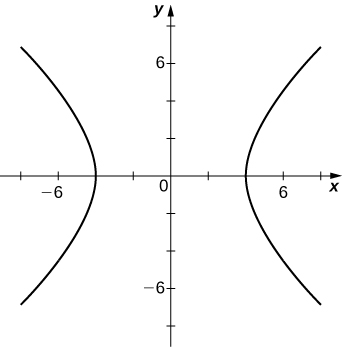$x=8$

For the following exercises, convert the rectangular equation to polar form and sketch its graph.

$3x-y=2$

$r=\frac{2}{3\phantom{\rule{0.2em}{0ex}}\text{cos}\phantom{\rule{0.2em}{0ex}}\theta -\text{sin}\phantom{\rule{0.2em}{0ex}}\theta }$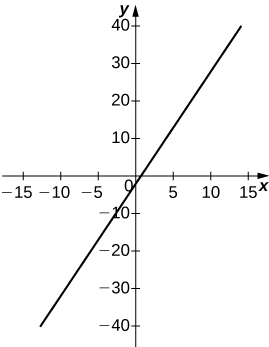${y}^{2}=4x$

For the following exercises, convert the polar equation to rectangular form and sketch its graph.

$r=4\phantom{\rule{0.2em}{0ex}}\text{sin}\phantom{\rule{0.2em}{0ex}}\theta$

${x}^{2}+{y}^{2}=4y$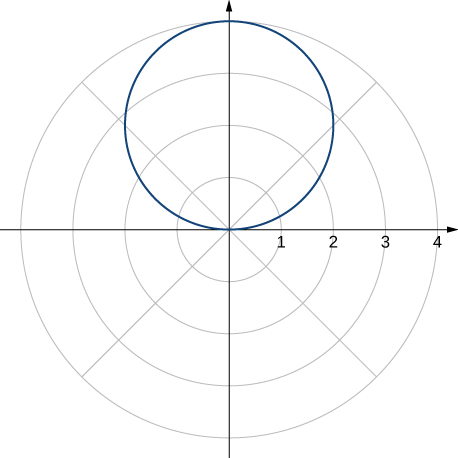$r=6\phantom{\rule{0.2em}{0ex}}\text{cos}\phantom{\rule{0.2em}{0ex}}\theta$

$r=\theta$

$x\phantom{\rule{0.2em}{0ex}}\text{tan}\sqrt{{x}^{2}+{y}^{2}}=y$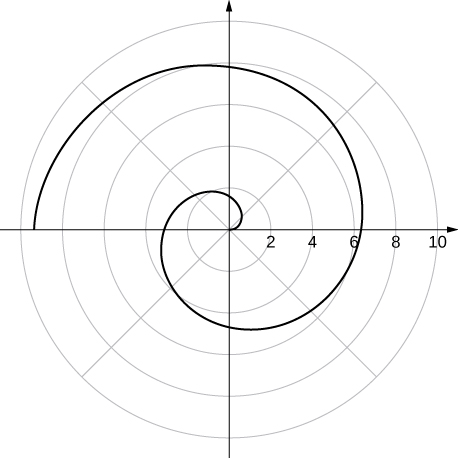$r=\text{cot}\phantom{\rule{0.2em}{0ex}}\theta \phantom{\rule{0.2em}{0ex}}\text{csc}\phantom{\rule{0.2em}{0ex}}\theta$

For the following exercises, sketch a graph of the polar equation and identify any symmetry.

$r=1+\text{sin}\phantom{\rule{0.2em}{0ex}}\theta$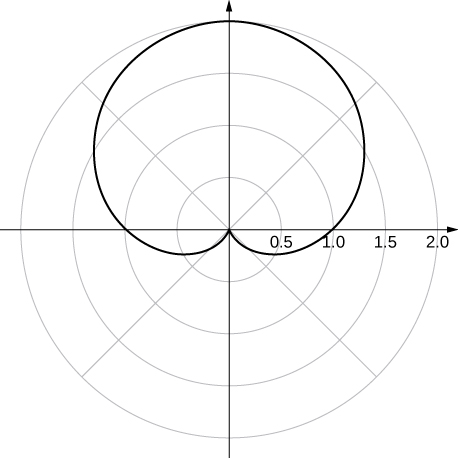y -axis symmetry

$r=3-2\phantom{\rule{0.2em}{0ex}}\text{cos}\phantom{\rule{0.2em}{0ex}}\theta$

$r=2-2\phantom{\rule{0.2em}{0ex}}\text{sin}\phantom{\rule{0.2em}{0ex}}\theta$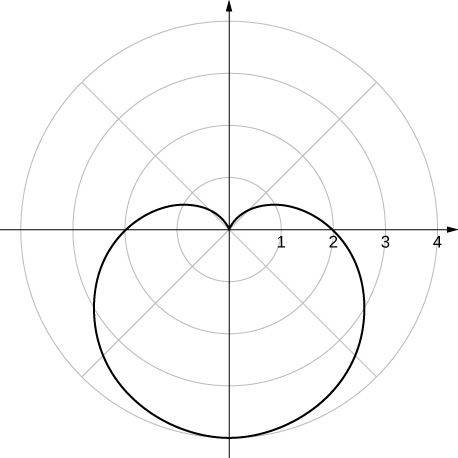y -axis symmetry

$r=5-4\phantom{\rule{0.2em}{0ex}}\text{sin}\phantom{\rule{0.2em}{0ex}}\theta$

$r=3\phantom{\rule{0.2em}{0ex}}\text{cos}\left(2\theta \right)$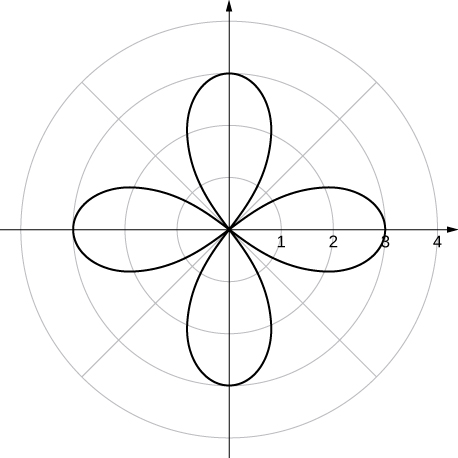x - and y -axis symmetry and symmetry about the pole

$r=3\phantom{\rule{0.2em}{0ex}}\text{sin}\left(2\theta \right)$

$r=2\phantom{\rule{0.2em}{0ex}}\text{cos}\left(3\theta \right)$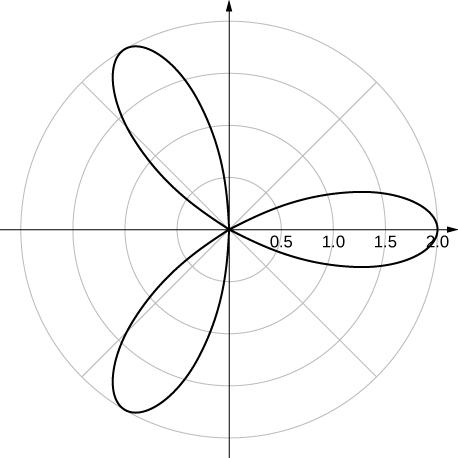x -axis symmetry

$r=3\phantom{\rule{0.2em}{0ex}}\text{cos}\left(\frac{\theta }{2}\right)$

${r}^{2}=4\phantom{\rule{0.2em}{0ex}}\text{cos}\left(2\theta \right)$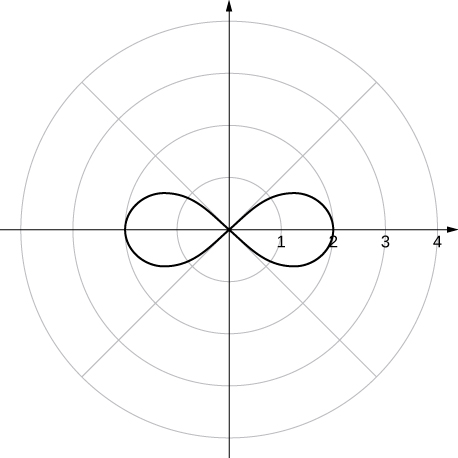x - and y -axis symmetry and symmetry about the pole

${r}^{2}=4\phantom{\rule{0.2em}{0ex}}\text{sin}\phantom{\rule{0.2em}{0ex}}\theta$

$r=2\theta$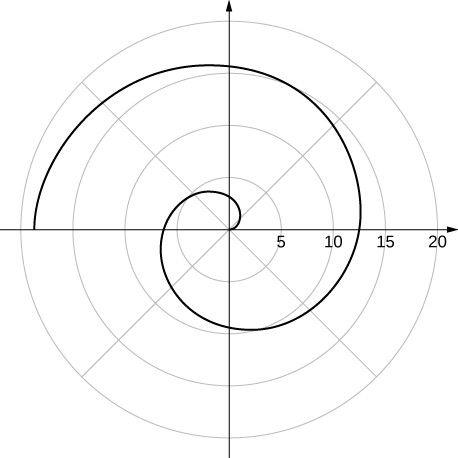no symmetry

[T] The graph of $r=2\phantom{\rule{0.2em}{0ex}}\text{cos}\left(2\theta \right)\text{sec}\left(\theta \right).$ is called a strophoid. Use a graphing utility to sketch the graph, and, from the graph, determine the asymptote.

[T] Use a graphing utility and sketch the graph of $r=\frac{6}{2\phantom{\rule{0.2em}{0ex}}\text{sin}\phantom{\rule{0.2em}{0ex}}\theta -3\phantom{\rule{0.2em}{0ex}}\text{cos}\phantom{\rule{0.2em}{0ex}}\theta }.$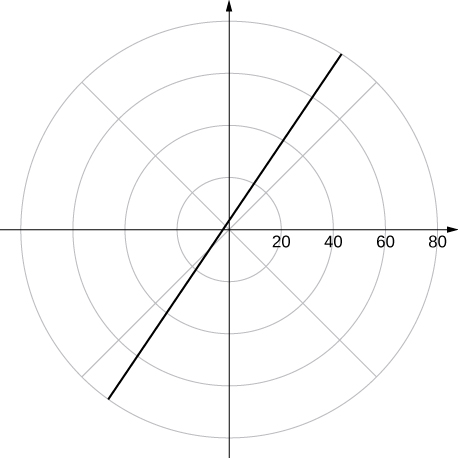a line

[T] Use a graphing utility to graph $r=\frac{1}{1-\text{cos}\phantom{\rule{0.2em}{0ex}}\theta }.$

[T] Use technology to graph $r={e}^{\text{sin}\left(\theta \right)}-2\phantom{\rule{0.2em}{0ex}}\text{cos}\left(4\theta \right).$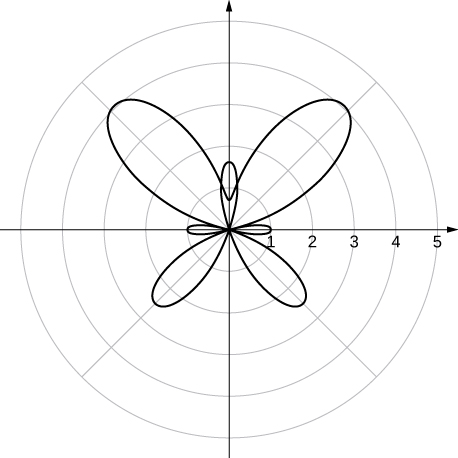[T] Use technology to plot $r=\text{sin}\left(\frac{3\theta }{7}\right)$ (use the interval $0\le \theta \le 14\pi \right).$

Without using technology, sketch the polar curve $\theta =\frac{2\pi }{3}.$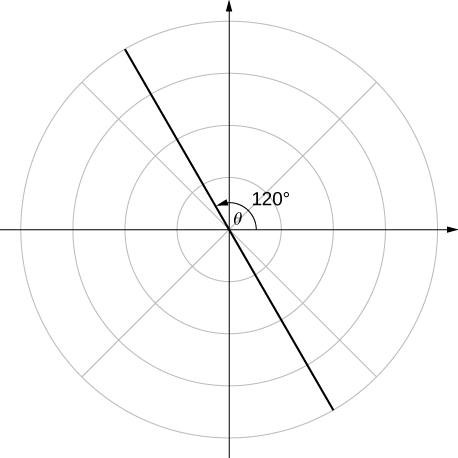[T] Use a graphing utility to plot $r=\theta \phantom{\rule{0.2em}{0ex}}\text{sin}\phantom{\rule{0.2em}{0ex}}\theta$ for $\text{−}\pi \le \theta \le \pi .$

[T] Use technology to plot $r={e}^{-0.1\theta }$ for $-10\le \theta \le 10.$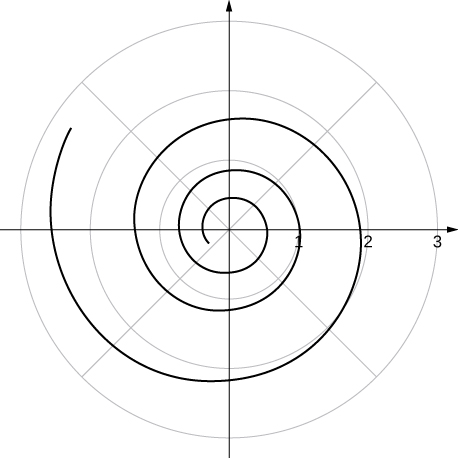[T] There is a curve known as the “ Black Hole .” Use technology to plot $r={e}^{-0.01\theta }$ for $-100\le \theta \le 100.$

[T] Use the results of the preceding two problems to explore the graphs of $r={e}^{-0.001\theta }$ and $r={e}^{-0.0001\theta }$ for $|\theta |>100.$

Answers vary. One possibility is the spiral lines become closer together and the total number of spirals increases.

what is the stm
is there industrial application of fullrenes. What is the method to prepare fullrene on large scale.?
Rafiq
industrial application...? mmm I think on the medical side as drug carrier, but you should go deeper on your research, I may be wrong
Damian
How we are making nano material?
what is a peer
What is meant by 'nano scale'?
What is STMs full form?
LITNING
scanning tunneling microscope
Sahil
how nano science is used for hydrophobicity
Santosh
Do u think that Graphene and Fullrene fiber can be used to make Air Plane body structure the lightest and strongest. Rafiq
Rafiq
what is differents between GO and RGO?
Mahi
what is simplest way to understand the applications of nano robots used to detect the cancer affected cell of human body.? How this robot is carried to required site of body cell.? what will be the carrier material and how can be detected that correct delivery of drug is done Rafiq
Rafiq
what is Nano technology ?
write examples of Nano molecule?
Bob
The nanotechnology is as new science, to scale nanometric
brayan
nanotechnology is the study, desing, synthesis, manipulation and application of materials and functional systems through control of matter at nanoscale
Damian
Is there any normative that regulates the use of silver nanoparticles?
what king of growth are you checking .?
Renato
What fields keep nano created devices from performing or assimulating ? Magnetic fields ? Are do they assimilate ?
why we need to study biomolecules, molecular biology in nanotechnology?
?
Kyle
yes I'm doing my masters in nanotechnology, we are being studying all these domains as well..
why?
what school?
Kyle
biomolecules are e building blocks of every organics and inorganic materials.
Joe
anyone know any internet site where one can find nanotechnology papers?
research.net
kanaga
sciencedirect big data base
Ernesto
Introduction about quantum dots in nanotechnology
what does nano mean?
nano basically means 10^(-9). nanometer is a unit to measure length.
Bharti
do you think it's worthwhile in the long term to study the effects and possibilities of nanotechnology on viral treatment?
absolutely yes
Daniel
how to know photocatalytic properties of tio2 nanoparticles...what to do now
it is a goid question and i want to know the answer as well
Maciej
Abigail
for teaching engĺish at school how nano technology help us
Anassong
How can I make nanorobot?
Lily
Do somebody tell me a best nano engineering book for beginners?
there is no specific books for beginners but there is book called principle of nanotechnology
NANO
how can I make nanorobot?
Lily
what is fullerene does it is used to make bukky balls
are you nano engineer ?
s.
fullerene is a bucky ball aka Carbon 60 molecule. It was name by the architect Fuller. He design the geodesic dome. it resembles a soccer ball.
Tarell
what is the actual application of fullerenes nowadays?
Damian
That is a great question Damian. best way to answer that question is to Google it. there are hundreds of applications for buck minister fullerenes, from medical to aerospace. you can also find plenty of research papers that will give you great detail on the potential applications of fullerenes.
Tarell
Difference between extinct and extici spicies
Leaves accumulate on the forest floor at a rate of 2 g/cm2/yr and also decompose at a rate of 90% per year. Write a differential equation governing the number of grams of leaf litter per square centimeter of forest floor, assuming at time 0 there is no leaf litter on the ground. Does this amount approach a steady value? What is that value?
You have a cup of coffee at temperature 70°C, which you let cool 10 minutes before you pour in the same amount of milk at 1°C as in the preceding problem. How does the temperature compare to the previous cup after 10 minutes?
AbdulByByByByBy